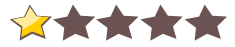# F.E.A.R.For: F.E.A.R.
 Rate this cheat:
Viewed 1571 times
F.E.A.R. cheats, F.E.A.R. code, F.E.A.R. cheat, F.E.A.R. combo, F.E.A.R. cheat code, F.E.A.R. gameplay cheats
free F.E.A.R. cheat, F.E.A.R., F.E.A.R. free combos, F.E.A.R. gaming combo, F.E.A.R. level cheats, F.E.A.R. help codes
No comments. Comment to start the discussion!